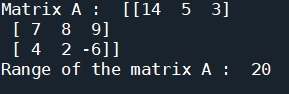# Python | Range of a Matrix

Python | Range of a Matrix: Here, we are going to learn about the range of a matrix and how to find it?
Submitted by Anuj Singh, on July 11, 2020

The range of a matrix can be defined as the difference between the maximum and minimum among the elements of the matrix. In NumPy, we have provided with an inbuilt function for this operation i.e. numpy.ptp(). It returns the range of the matrix by calculating maximum-minimum.## Python code to find range of a matrix

```# Linear Algebra Learning Sequence
# Range of a Matrix

import numpy as np

a = np.array([[14,5,3], [7,8,9], [4,2,-6]])

print('Matrix A : ', a)

# Range
print('Range of the matrix A : ', np.ptp(a))
```

Output:

```Matrix A :  [[14  5  3]
[ 7  8  9]
[ 4  2 -6]]
Range of the matrix A :  20
```

Preparation

What's New

Top Interview Coding Problems/Challenges!